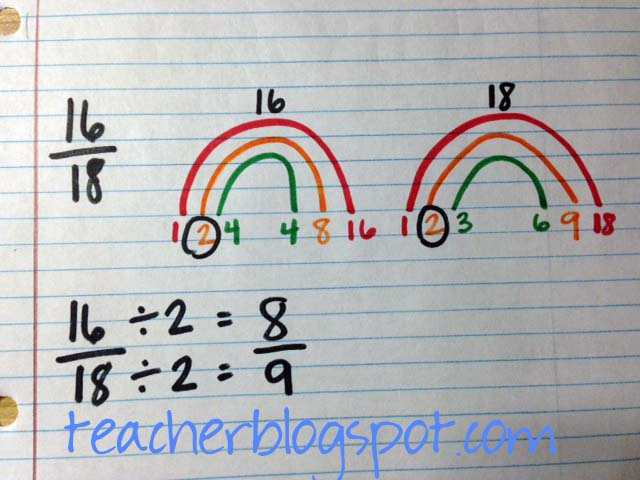Great Math Products!

# Simplify Fractions Using These

I must have found this idea on Pinterest somewhere…I would give credit if I remembered where, but it works so well!  I love it–Factoring Rainbows!  About 2 weeks ago I used this with the fifth graders who were struggling with simplifying fractions.  Using this trick really helped them.  Below you can see how it works.There is a rainbow for both the numerator and the denominator.   The rainbows always start with 1 and the number itself on the first arch.  Then each arch underneath progresses with the next largest factor.  With the 15 rainbow, 2 was not a factor, so I skipped to 3.  Three and 5 are factor pairs.  I could then try the factor of 4, but the only other number that could be a factor would be 4 itself.   I know 4 x 4 is 16, so that won’t make 15.  Then I could move on to a factor of 5, but I know that 5 has already been used.  When the factors repeat in the rainbow, then I know I have found all of the factors.  To simplify fractions, circle the largest common number in both rainbows  (GCF) and divide both the numerator and denominator by the largest common factor.Here is another example above.  I factored both the numerator–16 and the denominator–18.  I started the factor rainbow of 16 with an arch having the factor pair 1 and 16.  Then I moved on to an arch with the factor pair 2 and 8.  Three won’t equally divide into 16, so 3 is skipped.  Next, I know 4 and 4 will multiply to make 16.  Because these numbers repeat, I know the 16 factor rainbow is complete.  The factor rainbow of 18 begins with the first arch’s factor pair 1 and 18.  Next 2 and 9 are a factor pair, followed by 3 and 6.  Then, I ask myself if 4 would be a factor.  What about 5? Nope.  So that brings me back to 6.  I know that I have found all of the factor pairs because I have reached a repeating factor–6.  Then I circle the greatest common factor of 2 and divide the numerator and denominator by 2.If a fraction is already simplified, the fraction rainbows will help show this, too.  The only thing that will be different is that the largest common factor will be a 1.  Dividing by 1 doesn’t change the numerator or denominator obviously so the fraction is already simplified.

•